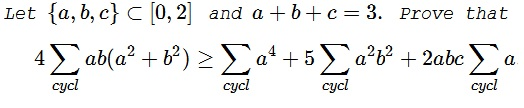# Michael Rozenberg's Inequality in Three Variables with Constraints

### Problem### Solution 1

The essential fact of the proof is to observe that $2\le ab+bc+ca\le 3.\,$ Indeed,

$3(ab+bc+ca)\le (a+b+c)^2\,\Rightarrow\,ab+bc+ca\le 3.$

Let $a=\max\{a,b,c\}.\,$ Then $a\in [1,2],\,$ so that

\begin{align} ab+bc+ca&=a(b+c)+bc=a(3-a)+bc\\ &\ge 2+bc\ge 2. \end{align}

After usual algebraic manipulations, the required inequality is equivalent to

(*)

$\displaystyle 12\sum_{cycl}a^3\ge5\left(\sum_{cycl}a^2\right)^2-5\sum_{cycl}a^2b^2+6abc.$

From the above, $ab+bc+ca=3(1-t),\,$ with $t\in\left[0,\frac{1}{3}\right].\,$ With this in mind,

\begin{align} &a^3+b^3+c^3=3(9t+abc)\\ &a^2+b^2+c^2=3(1+2t)\\ &a^2b^2+b^2c^2+c^2a^2=9(1-t)^2-6abc. \end{align}

Thus, (*) can be rewritten as

$36(9t+abc)\ge 45(1+2t)^2-45(1-t)^2+36abc,$

or, equivalently, $36t\ge 5(1+2t)^2-5(1-t)^2,\,$ i.e., $2t\ge 25t^2,\,$ which is true because $t\in\left[0,\frac{1}{3}\right].$

### Solution 2

Rewriting

$\left(a^2 +b^2 +c^2 -3 a b+3 a c-3 b c\right) \left(a^2+b^2+c^2-a b-\text{ac}-b c\right)\leq 0$

By Schur's inequality: $\left(a^2+b^2+c^2-a b-\text{ac}-b c\right)\geq 0$. So we need to prove that

$g=\left( \underbrace{(a^2 +b^2 +c^2)}_{\lambda_1}-3\underbrace{(a b+ a c+ b c)}_{\lambda_2} \right) \leq 0.$

$\lambda_1$ is convex, $\lambda_2$ is concave. So the maximum of $\lambda_1=5$ is reached (under the constraint that $a+b+c=3$) at the maximum dispersion, that is $\{2,1,0\}$. The same for the minimum of $\lambda_2=6$. Hence $g\leq -1$.### Acknowledgment

I am grateful to Leo Giugiuc for kindly communicating to me the above problem by Michael Rozenberg (Tel-Aviv, Israel), along with his solution. Solution 2 is by N. N. Taleb.